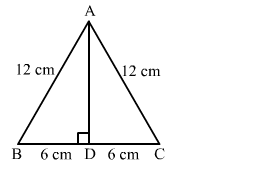# The height of an equilateral triangle having each side 12 cm, is

Question:

The height of an equilateral triangle having each side 12 cm, is

(a) $6 \sqrt{2} \mathrm{~cm}$

(b) $6 \sqrt{3} \mathrm{~cm}$

(c) $3 \sqrt{6} \mathrm{~cm}$

(d) $6 \sqrt{6} \mathrm{~cm}$

Solution:

(b) $6 \sqrt{3} \mathrm{~cm}$Let ABC be the equilateral triangle with AD as its altitude from A.

In right-angled triangle ABD, we have:

$A B^{2}=A D^{2}+B D^{2}$

$A D^{2}=A B^{2}-B D^{2}$

$=12^{2}-6^{2}$

$=144-36=108$

$A D=\sqrt{108}=6 \sqrt{3} \mathrm{~cm}$